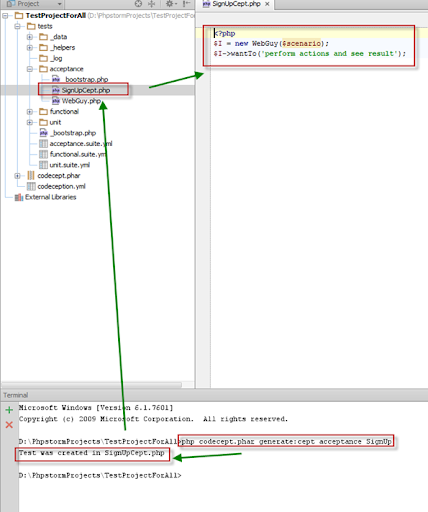In this article we are going to see How can we create Cept test case in codeception?
As we know , codeception has 3 type of tests, we have to define what type of test we are going to build.
So, what is Cept format ?
In codeception, we use BDD type test steps to write test case. What is BDD type? BDD refers to Behavior Driven Development. BDD type test first introduced in Gherkin with Cucumber. But in here, we will write similar test cases so, we are referring that as BDD type test case.
In codeception, when we need to declare BDD type test case, we need to use Cept as post fix of a test which is understand by codeception command. That means, if our test case name is SugnUp, so if we are writing BDD type test case, then it will be named as SignUpCept.php.

Cept Format :
This type of test case start with Guy ( either WebGuy/ TestGuy/ TestGuy).
Like :
\$I = new WebGuy(\$scenario);
\$I->wantTo('travelling through site');
\$I->amOnPage('/”);

So, now lets move on to command. When we need to generate a Cept format Acceptance test case(acceptance is a type of test suit) :

php codecept.phar generate:cept <suitename> <testname>

[if you use composer, then : <path to codecept>/codecept generate:cept <suitename> <testname> ]

For example, if we want to generate an Acceptance test case named as SignUp in cept format , then

php codecept.phar generate:cept acceptance SignUpThen,  a file will be create SignUpCept.php in the tests/acceptance/ folder and it will follow the configuration of acceptance.suite.yml

So, we can generate cept format test case and we can ready to write codes.

Thanks…:)